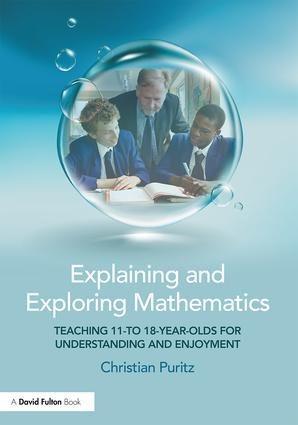# Explaining and Exploring Mathematics

## Teaching 11- to 18-year-olds for understanding and enjoyment, 1st Edition

Routledge

218 pages | 75 B/W Illus.

Paperback: 9781138680210
pub: 2017-01-27
SAVE ~\$7.79
\$38.95
\$31.16
x
Hardback: 9781138680197
pub: 2017-01-27
SAVE ~\$35.00
\$175.00
\$140.00
x
eBook (VitalSource) : 9781315563688
pub: 2017-04-28
from \$19.48

FREE Standard Shipping!

### Description

Explaining and Exploring Mathematics is designed to help you teach key mathematical concepts in a fun and engaging way by developing the confidence that is vital for teachers. This practical guide focuses on improving students’ mathematical understanding, rather than just training them for exams. Covering many aspects of the secondary mathematics curriculum for ages 11-18, it explains how to build on students’ current knowledge to help them make sense of new concepts and avoid common misconceptions.

Focusing on two main principles to improve students’ understanding: spotting patterns and extending them to something new, and relating the topic being taught to something that the pupils already understand, this book helps you to explore mathematics with your class and establish a successful teacher-student relationship.

Structured into a series of lessons, Explaining and Exploring Mathematics is packed full of practical advice and examples of the best way to answer frequently asked questions such as:

• Do two minuses really make a plus?
• Why doesn’t 3a + 4b equal 7ab?
• How do you get the area of a circle?
• Why do the angles of a triangle add up to 180°?
• How can you integrate 1/x and calculate the value of e?

This book will be essential reading for all trainee and practising teachers who want to make mathematics relevant and engaging for their students.

Introduction Part I: 11-14 years old 1. Decimals and multiplication by 10 etc. 2. Multiplying and dividing by decimals 3. Adding fractions 4. Multiplying and dividing by fractions; and by 0? 5. Using patterns with negative numbers 6. Use hundreds and thousands, not apples and bananas! 7. Angles and polygons 8. Special quadrilaterals 9. Basic areas 10. Circles and pi 11. Starting trigonometry 12. Square of a sum and sum of squares 13. The difference of two squares 14. Another look at (a-b)(a+b) 15. Number museum: how many factors? Part II: 14-16 years old 1. The difference of two squares revisited 2. The m,d method: an alternative approach to quadratics 3. Negative and fractional indices 4. A way to calculate pi 5. Pyramids and cones 6. Volume and area of a sphere 7. Straight line graphs 8. Percentage changes 9. Combining small percentage changes 10. Trigonometry with general triangles 11. Irrational numbers 12. Minimising via reflection 13. Maximum area for given perimeter 14. Farey sequences 15. Touching circles & Farey sequences again Part III: 16-18 years old 1. Remainder theorem… 2. Adding arithmetic series 3. D why? by dx; or What is differentiation for? 4. Integration without calculus 5. Integration using calculus 6. Summing series: using differencing instead of induction 7. Geometric series, perfect numbers and repaying a loan 8. Binomial expansion and counting 9. How to make your own logarithms 10. The mysterious integral of 1/x 11. Differentiating exponential functions 12. Why do the trig ratios have those names? 13. Compound angle formulae 14. Differentiating trig ratios 15. Fermat centre of a triangle

#### Christian Wilhelm Puritz

High Wycombe, Bucks, UK